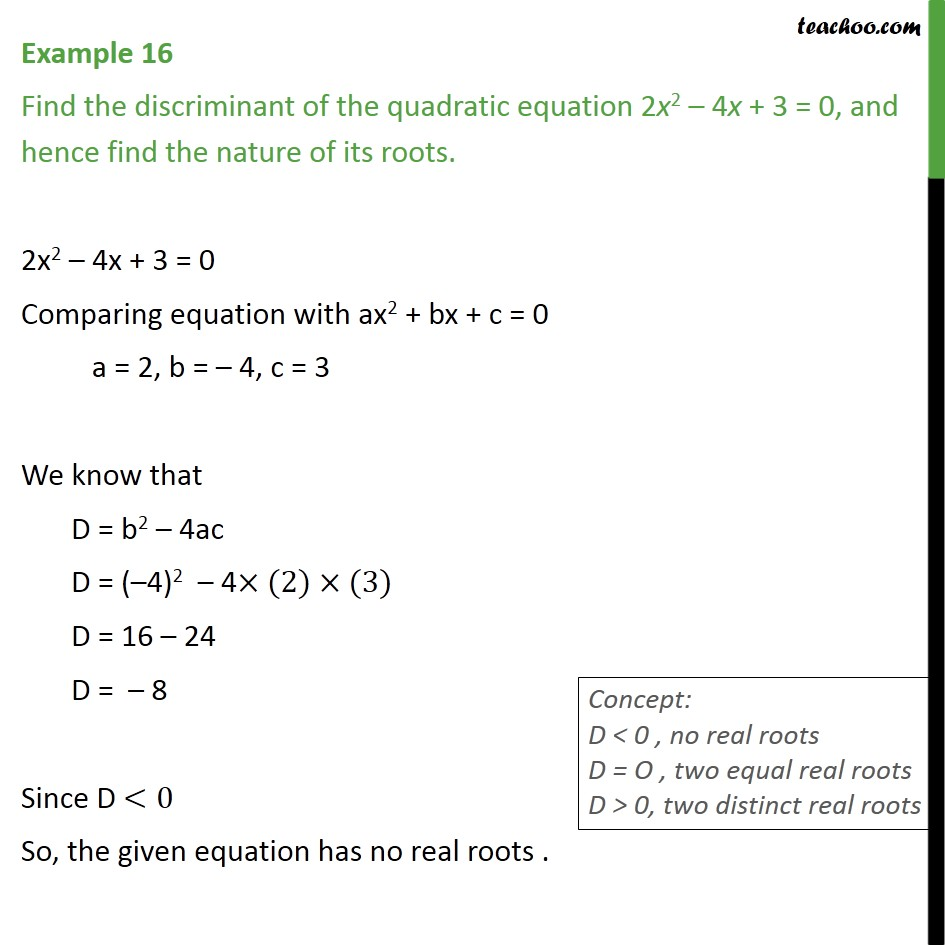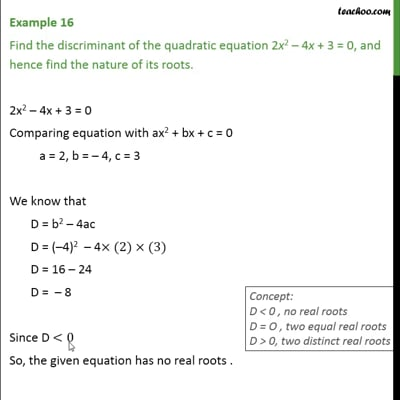Examples

Chapter 4 Class 10 Quadratic Equations
Serial order wiseThis video is only available for Teachoo black users

Maths Crash Course - Live lectures + all videos + Real time Doubt solving!

### Transcript

Example 16 Find the discriminant of the quadratic equation 2x2 4x + 3 = 0, and hence find the nature of its roots. 2x2 4x + 3 = 0 Comparing equation with ax2 + bx + c = 0 a = 2, b = 4, c = 3 We know that D = b2 4ac D = ( 4)2 4 (2) (3) D = 16 24 D = 8 Since D <0 So, the given equation has no real roots .